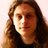## Map Reduce for Mortals [4800 views]

Suppose you want the sum of the squares of the  elements of a list congruent to 1 mod 3 you can write

reduce(lambda t,x t+x,map(square,filter(lambda x: x % 3 == 1,[1,2,3,4,5])))

Clear? As mud … (there are plenty of tutorials online about map, filter and reduce).

You can always write a loop in an imperative language

ssq=0
for i in [1,2,3,4,5]:

if i % 3 == 1 :
ssq += i**2

Which is better? Hard to say. But the reduce version is declarative and nonsequential: there is no specified order in which the operations are to be performed, especially if we take into account the fact that addition is associative.On the other hand, the lambdas and the functions obscure what is being computed. We need a notation that is clear but not imperative. Enter Variable Binding Operators (VBOS).

VBOs are inspired by conventional mathematical notation, which has been honed over centuries to be both concise and readable. A mathematician would write

∑ i2
i∈[1,5]
i mod 3 = 1

(that’s as close as I can get in wordpress but you know what it looks like).

The higher order functions map, reduce, and filter are nowhere to be seen, nor is lambda. This is a first order expression and as a  result is much easier for mere mortals to comprehend. My suggestion is to make something like it available in, say, pyLucid.

What form should it take? Two dimensional syntax is impractical and for a lot of reasons it’s best to stick with ASCII characters. So we need a linear ASCII expression that incorporates the variable varying over a range, the range, the filtering condition, and the expression being summed. Here is what I propose:

sumfor i in [1,2,3,4,5]: i % 3 = 1 all i**2 end

If there is no condition we just omit it:

sumfor i in [1,2,3,4,5] all i**2 end

If we want the product, we use prodfor;

prodfor i in [1,2,3,4,5]: i % 3 = 1 all i**2 end

If we want the average we use avgfor; if we want to accumulate concatenating strings we use concatfor. You can surely guess the semantics of maxfor, minfor, appendfor, consfor, unionfor, and intersectfor.

There are also VBOs that don’t follow this pattern (because they don’t have an implied reduce): the meanings of first i in R: i>0, last i in R: i>0, exist i in R: i>0, forall i in R: i>0, those i in R: i>0  should be clear. Notice that those and consfor are list comprehensions with a  different syntax.

Here are is the primes less than 100

those p in range(2,100):
forall d  in range (2,round(sqrt(p))+1):
p mod d ne 0
end
end

Here is a definition of a function perms that returns a list of all permutations of its argument, in hypothetical extended pyLucid:

perms(L) =
appendfor i in L all
consfor  J in perms(those j in L: j ne i end) all
i :: J
end
end

And here is a definition of the list of sublists of a list L

sublists(L) =
if L eq [] then [] else
sublists(tl(L)) <>
appendfor J in sublists(tl(L))  all
hd(L) :: J
end
fi

With the VBOs we can simply transcribe many mathematical formulas. Here is e**x approximated by the first 10 terms of the power series:

sumfor n in range(10) all
prodfor i in range(n) all
x/(i+1)

Of course this straight-from-the-book expression is inefficient but at least we’re sure that it’s right.

One simple extension is to allow to bound variables running in parallel over different ranges; for example

sumfor a,b in A,B all a*b end

computes a dot product.

We could also have a VBO that extracts the ‘final’ value from a converging series:

limit n in range(1,100) all (1+1/n)**n end

(which assumes some otherwise specified convergence criterion).

Great … so pyLucid has VBOS. Sadly, not yet (and these examples haven’t been tested). There are problems.

The first problem is that every VBO is an implied loop. PyLucid already has (nested) loops so how do they interact? The obvious implementation technique is to translate VBOs into nested loops but I haven’t figured out the details.

A more difficult question is, what sort of objects are ranges? In Python they would be iterators, and that works out fine. In Haskell they would be lists.

I think the best choice is to make them vectors (arrays) but what if the other expressions also vary in space? Do we need extra space dimensions?

For once I’m stumped. I’ll let you know when I get on top of it all.I am a retired Professor in Computer Science at UVic.
This entry was posted in Uncategorized. Bookmark the permalink.

### 3 Responses to Map Reduce for Mortals [4800 views]

1.eschew says:

Even without using syntax sugar for list comprehensions, your original version with explicit map and filter becomes much more readable with the use of a pipeline-style operator as found in many languages:

[1,2,3,4,5]
| filter (\x .x % 3 == 1)
| map square
| sum

2.Matt S Trout, 43*47 Edition (@shadowcat_mst) says:

I’ve been experimenting with code that provides both pipeline and method-style – and did in fact start with pipeline style before your comment and that worked fine, but I think I finally prefer

(1, 2, 3, 4, 5).where{this.mod 3 == 1}.map{this**2}.reduce 0 +;

(why the explicit zero? because in my current experiments ints and floats don’t talk to each other without explicitly asking for that so you need to seed the reduce with 0 or 0.0 to tell + which one it’s working on … this may or may not turn out to have been a good idea)

This site uses Akismet to reduce spam. Learn how your comment data is processed.